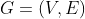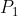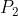Warning: count(): Parameter must be an array or an object that implements Countable in /home/customer/www/thegatebook.in/public_html/qa/qa-include/qa-theme-base.php on line 177

# DSA - Graphs and Hashing -Q2

Warning: count(): Parameter must be an array or an object that implements Countable in /home/customer/www/thegatebook.in/public_html/qa/qa-include/qa-theme-base.php on line 177
+1 vote

Letbe a connected, undirected graph, and let a and b be two distinct vertices in V. Letbe the problem of finding a shortest simple path between a and b, and letbe the problem of finding a longest simple path between a and b.

Which of the following statements aboutandis true?

(A) Bothandcan be solved in polynomial time.

(B)can be solved in polynomial time butis not known to be solvable in polynomial time.

(C)is not known to be solvable in polynomial time butcan be solved in polynomial time.

(D) It is not known whether eitherorcan be solved in polynomial time.asked Aug 3, 2020
reshown Aug 6, 2020

+1 vote

The longest path problem for a general graph is not as easy as the shortest path problem because the longest path problem doesn&rsquo;t have optimal substructure property. In fact, the Longest Path problem is NP-Hard for a general graph.

https://www.quora.com/Why-is-the-longest-path-problem-NP-complete-given-that-the-shortest-path-problem-is-not-NP-completeanswered Aug 14, 2020 by (226,240 points)
It would be better if in Answer you tell why it is NP hard. :)

We all know P1 is nlogn.
But P2 is NP complete.
Given a solution, you can verify it. But it is not NP hard bro.
Every NP complete is NP hard.
And telling why a problem is NP hard would take around 8-10 pages of answer.
This question could be skipped as well as NP complexity is not in syllabus.